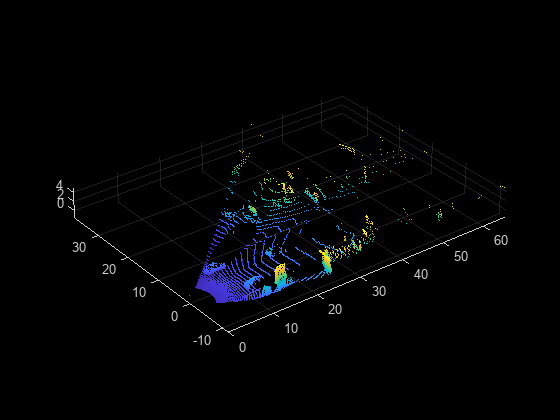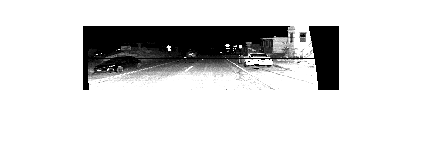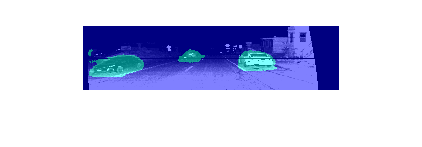# pcsemanticseg

Point cloud semantic segmentation using deep learning

Since R2022b

## Syntax

``C = pcsemanticseg(pc,network)``
``[C,score,allScores] = pcsemanticseg(pc,network)``
``plds = pcsemanticseg(ds,network)``
``[___] = pcsemanticseg(___,Name=Value)``

## Description

example

````C = pcsemanticseg(pc,network)` performs the semantic segmentation results on the input point cloud using deep learning and returns the results.```
````[C,score,allScores] = pcsemanticseg(pc,network)` additionally returns the classification score `score` for each predicted label in `C` and the scores of all the label categories that the network can classify `allScores`.```

example

````plds = pcsemanticseg(ds,network)` returns the semantic segmentation results for a collection of point clouds in a datastore object `ds`.The function supports parallel computing using multiple MATLAB® workers. You can enable parallel computing using the Computer Vision Toolbox Preferences dialog box.```
````[___] = pcsemanticseg(___,Name=Value)` specifies options using one or more name-value arguments. For example, `OutputType="double"` returns the segmentation results as numeric array of data type `double`.```

## Examples

collapse all

Load a pretrained network into the workspace. This network segments vehicles from the input point cloud.

```data = load("pointCloudVehicleSegmentationNetwork.mat"); net = data.net```
```net = DAGNetwork with properties: Layers: [80×1 nnet.cnn.layer.Layer] Connections: [87×2 table] InputNames: {'Organized-Point-Cloud-Input'} OutputNames: {'Loss'} ```

Load the test point cloud data.

```dataDir = fullfile(toolboxdir("lidar"),"lidardata", ... "sampleWPIPointClouds","pointClouds","010.pcd");```

Read and display the test point cloud.

```ptCloud = pcread(dataDir); figure pcshow(ptCloud.Location)```Display the intensity channel of the point cloud.

```figure imshow(uint8(ptCloud.Intensity))```Preprocess the point cloud data and perform semantic segmentation.

```pc = cat(3,ptCloud.Location,ptCloud.Intensity); [C,scores] = pcsemanticseg(pc,net);```

Overlay the segmentation results on the intensity channel and display the results.

```B = labeloverlay(uint8(ptCloud.Intensity),C); figure imshow(B)```Load a pretrained network into the workspace. This network segments vehicles from the input point cloud.

```data = load("pointCloudVehicleSegmentationNetwork.mat"); net = data.net;```

Load the test point cloud data, and create a file datastore.

```dataDir = fullfile(toolboxdir("lidar"),"lidardata","sampleWPIPointClouds"); testDataDir = fullfile(dataDir,"pointClouds"); ptCloudDs = fileDatastore(testDataDir,ReadFcn=@pcread);```

Concatenate the intensity channel values to the datastore.

`pcds = transform(ptCloudDs,@(ptCloud)cat(3,ptCloud.Location,ptCloud.Intensity));`

```testLabelDir = fullfile(dataDir,"segmentationLabels"); pldsTruth = fileDatastore(testLabelDir,ReadFcn=@(x)load(x).labels);```

Perform semantic segmentation on all the test point clouds.

`pldsResults = pcsemanticseg(pcds,net,WriteLocation=tempdir);`
```Running semantic segmentation network ------------------------------------- * Processed 10 point clouds. ```

Compare the results against the ground truth labels.

`metrics = evaluateSemanticSegmentation(pldsResults,pldsTruth)`
```Evaluating semantic segmentation results ---------------------------------------- * Selected metrics: global accuracy, class accuracy, IoU, weighted IoU, BF score. * Processed 10 images. * Finalizing... Done. * Data set metrics: GlobalAccuracy MeanAccuracy MeanIoU WeightedIoU MeanBFScore ______________ ____________ _______ ___________ ___________ 0.94751 0.9723 0.67226 0.92655 0.47675 ```
```metrics = semanticSegmentationMetrics with properties: ConfusionMatrix: [2×2 table] NormalizedConfusionMatrix: [2×2 table] DataSetMetrics: [1×5 table] ClassMetrics: [2×3 table] ImageMetrics: [10×5 table] ```

## Input Arguments

collapse all

Input point cloud, specified as one of these options.

Point Cloud TypeData Format
Unorganized point cloudNumeric matrix of size M-by-K, where M is the number of points in the point cloud, and K is the number of channels, such as intensity and color.
Array of unorganized point clouds3-D numeric array of size M-by-K-by-P, where P is the number of point clouds.
Organized point cloud3-D numeric array of size M-by-N-by-K, where M and N are the rows and columns in the point cloud, respectively, and K is the number of channels, such as intensity and color.
Array of organized point clouds4-D numeric array of size M-by-N-by-K-by-P, where P is the number of point clouds.

The input point cloud can also be a `gpuArray` (Parallel Computing Toolbox) that contains one of the point cloud types listed in the table (requires Parallel Computing Toolbox™).

Data Types: `double` | `single`

Network, specified as a `SeriesNetwork` (Deep Learning Toolbox), `DAGNetwork` (Deep Learning Toolbox), or `dlnetwork` (Deep Learning Toolbox) object.

Collection of point clouds, specified as a datastore object. The `read` function of the datastore must return a numeric array or cell array. For a cell array, the data in each cell must be a numeric array. For cell arrays with multiple columns, the function processes only the first column.

### Name-Value Arguments

Specify optional pairs of arguments as `Name1=Value1,...,NameN=ValueN`, where `Name` is the argument name and `Value` is the corresponding value. Name-value arguments must appear after other arguments, but the order of the pairs does not matter.

Example: `pcsemanticseg(pc,network,OutputType="double")` returns the segmentation results as numeric array of data type `double`.

Returned segmentation type, specified as `"categorical"`, `"double"`, or `"uint8"`.

When you specify this value as `"categorical"`, the function returns the segmentation labels as a categorical array.

When you specify this value as `"double"` or `"uint8"`, the function returns the segmentation results as a numeric array of the corresponding data type containing label IDs. The IDs are integer values corresponding to the class names defined in the classification layer of the input network.

Note

You cannot use the `OutputType` argument with a datastore input.

Data Types: `char` | `string`

Size of the point cloud groups, specified as an integer. For a large collection of point clouds, the function groups and processes the point clouds together as a batch. Increasing the `MiniBatchSize` value improves the computational efficiency, but requires more memory.

Data Types: `single` | `double` | `int8` | `int16` | `int32` | `int64` | `uint8` | `uint16` | `uint32` | `uint64`

Hardware resource for processing the point clouds, specified as `"auto"`, `"gpu"`, or `"cpu"`.

Execution EnvironmentDescription
`"auto"`Use a GPU, if available. Otherwise, use the CPU. Using a GPU requires Parallel Computing Toolbox and a CUDA®-enabled NVIDIA® GPU. For information about the supported capabilities, see GPU Computing Requirements (Parallel Computing Toolbox).
`"gpu"`Use the GPU. If a suitable GPU is not available, the function returns an error message.
`"cpu"`Use the CPU.

Data Types: `char` | `string`

Performance optimization, specified as `"auto"`, `"mex"`, or `"none"`.

AccelerationDescription
`"auto"`

Automatically apply a number of optimizations suitable for the input network and hardware resource.

This is the default option. MATLAB does not generate a MEX function with this option.

`"none"`Disable all acceleration.

`"auto"` option can offer performance benefits, but at the expense of an increased initial run time. Subsequent calls with compatible parameters are faster. Use performance optimization when you plan to call the function multiple times using new input data.

Data Types: `char` | `string`

Classes into which points are classified, specified as `"auto"`, a cell array of character vectors, a vector of strings, or a categorical vector. If the value is a categorical vector, `Y`, then the function sorts and orders the elements of the vector according to `categories``(Y)`.

If the network is a `dlnetwork` (Deep Learning Toolbox) object, then the number of classes specified by `Classes` must match the number of channels in the output of the network predictions. By default, when the value of `Classes` is `"auto"`, the function numbers the classes from 1 through K, where K is the number of channels in the output layer of the network.

If the network is a `SeriesNetwork` (Deep Learning Toolbox) or `DAGNetwork` (Deep Learning Toolbox) object, then the number of classes specified by `Classes` must match the number of classes in the classification output layer. By default, when the value of `Classes` is `"auto"`, the function sets the classes automatically using the classification output layer.

Folder to write to, specified as `pwd`, a string scalar, or a character vector. The specified folder must exist and have write permissions.

Note

This argument is applicable only when you specify the input point clouds using a datastore.

Data Types: `char` | `string`

Prefix for the output file names, specified as a string scalar or character vector. The function returns the point cloud files as:

• `NamePrefix_N.png`, where `N` is the index of the corresponding point cloud file in `pointCloudDs.Files`.

Note

This argument is applicable only when you specify the input point clouds using a datastore.

Data Types: `char` | `string`

Display progress information, specified as logical `1` (`true`) or `0` (`false`).

Note

This argument is applicable only when you specify the input point clouds using a datastore.

Data Types: `logical`

Run parallel computations, specified as a logical `1` (`true`) or `0` (`false`).

To run in parallel, set `UseParallel` to `true`, or enable this by default using the Computer Vision Toolbox™ preferences.

Note

This argument is applicable only when you specify the input point clouds using a datastore.

Data Types: `logical`

## Output Arguments

collapse all

Segmentation labels, returned as a categorical array. The argument contains labels for all points in the input point cloud.

Input Point CloudSemantic Labels
Unorganized point cloudNumeric vector with M elements. Element `C`(i) is the categorical label assigned to the point `pc`(i) in the input point cloud.
Array of unorganized point cloudsNumeric matrix of size M-by-P. Element `C`(i,k) is the categorical label assigned to the ith point in the kth point cloud of the point cloud array.
Organized point cloudNumeric matrix of size M-by-N. Element `C`(i,j) is the categorical label assigned to the point `pc`(i,j) in the input point cloud.
Array of organized point clouds3-D numeric array of size M-by-N-by-P. Element `C`(i,j,k) is the categorical label assigned to the point `pc`(i,j) in the kth point cloud of the input point cloud array.

Confidence scores for each categorical label in `C`, returned as a vector, matrix, or array of values between `0` and `1`. The scores represents the confidence in the corresponding predicted label in `C`. Higher score values indicate a higher confidence in the predicted label.

Input Point CloudSemantic Labels
Unorganized point cloudNumeric vector with M elements. Element `score`(i) is the score assigned to the point `pc`(i) in the input point cloud.
Array of unorganized point cloudsNumeric matrix of size M-by-P. Element `score`(i,k) is the score assigned to the ith point in the kth point cloud of the point cloud array.
Organized point cloudNumeric matrix of size M-by-N. Element `score`(i,j) is the score assigned to the point `pc`(i,j) in the input point cloud.
Array of organized point clouds3-D numeric array of size M-by-N-by-P. Element `score`(i,j,k) is the score assigned to the point `pc`(i,j) in the kth point cloud of the input point cloud array.

Scores for all label categories the input network can classify, returned as a numeric array.

This table shows the format of this output for each type of point cloud input. L is the total number of label categories.

Input Point CloudSemantic Labels
Unorganized point cloudNumeric matrix of size M-by-L. Element `allScores`(i,q) is the score of the qth label at the point `pc`(i) in the input point cloud.
Array of unorganized point clouds3-D numeric array of size M-by-L-by-P. Element `allScores`(i,q,k) is the score of the qth label at the point `pc`(i) in the kth point cloud of the point cloud array.
Organized point cloud3-D numeric array of size M-by-N-by-L. Element `allScores`(i,j,q) is the score of the qth label at the point `pc`(i,j) in the input point cloud.
Array of organized point clouds4-D numeric array of size M-by-N-by-L-by-P. Element `allScores`(i,j,q,k) is the score of the qth label at the point `pc`(i, j) in the kth point cloud of the input point cloud array.

Semantic segmentation results, returned as a `fileDatastore` object. The function saves the segmentation result of each point cloud as a MAT file. You can use the `read` function on this output to obtain the categorical labels for the point clouds in `ds`.

## Version History

Introduced in R2022b